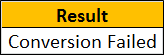Support us .Net Basics C# SQL ASP.NET ADO.NET MVC Slides C# Programs Subscribe Buy DVD

TRY_CONVERT function in SQL Server 2012

Suggested Videos
Part 121 - Choose function in SQL Server
Part 122 - IIF function in SQL Server
Part 123 - TRY_PARSE function in SQL Server 2012

In this video we will discuss
• TRY_CONVERT function
• Difference between CONVERT and TRY_CONVERT functions
• Difference between TRY_PARSE and TRY_CONVERT functions

TRY_CONVERT function
• Introduced in SQL Server 2012
• Converts a value to the specified data type
• Returns NULL if the provided value cannot be converted to the specified data type
• If you request a conversion that is explicitly not permitted, then TRY_CONVERT fails with an error
Syntax : TRY_CONVERT ( data_type, value, [style] )

Style parameter is optional. The range of acceptable values is determined by the target data_type. For the list of all possible values for style parameter, please visit the following MSDN article
https://msdn.microsoft.com/en-us/library/ms187928.aspx

Example : Convert string to INT. As the string can be converted to INT, the result will be 99 as expected.

SELECT TRY_CONVERT(INT, '99') AS Result

Output :Example : Convert string to INT. The string cannot be converted to INT, so TRY_CONVERT returns NULL

SELECT TRY_CONVERT(INT, 'ABC') AS Result

Output :Example : Converting an integer to XML is not explicitly permitted. so in this case TRY_CONVERT fails with an error

SELECT TRY_CONVERT(XML, 10) AS Result

If you want to provide a meaningful error message instead of NULL when the conversion fails, you can do so using CASE statement or IIF function.

Example : Using CASE statement to provide a meaningful error message when the conversion fails.

SELECT
CASE WHEN TRY_CONVERT(INT, 'ABC') IS NULL
THEN 'Conversion Failed'
ELSE 'Conversion Successful'
END AS Result

Output : As the conversion fails, you will now get a message 'Conversion Failed' instead of NULLExample : Using IIF function to provide a meaningful error message when the conversion fails.

SELECT IIF(TRY_CONVERT(INT, 'ABC') IS NULL, 'Conversion Failed',
'Conversion Successful') AS Result

What is the difference between CONVERT and TRY_CONVERT
CONVERT will result in an error if the conversion fails, where as TRY_CONVERT will return NULL instead of an error.

Since ABC cannot be converted to INT, CONVERT will return an error
SELECT CONVERT(INT, 'ABC') AS Result

Since ABC cannot be converted to INT, TRY_CONVERT will return NULL instead of an error
SELECT TRY_CONVERT(INT, 'ABC') AS Result

Example : Using TRY_CONVERT() function with table data. We will use the following Employees table for this example.SQL Script to create Employees table
Create table Employees
(
Id int primary key identity,
Name nvarchar(10),
Age nvarchar(10)
)
Go

Insert into Employees values ('Mark', '40')
Insert into Employees values ('John', '20')
Insert into Employees values ('Amy', 'THIRTY')
Insert into Employees values ('Ben', '21')
Insert into Employees values ('Sara', 'FIFTY')
Insert into Employees values ('David', '25')
Go

The data type of Age column is nvarchar. So string values like (THIRTY, FIFTY ) are also stored. Now, we want to write a query to convert the values in Age column to int and return along with the Employee name. Notice TRY_CONVERT function returns NULL for the rows where age cannot be converted to INT.

SELECT Name, TRY_CONVERT(INT, Age) AS Age
FROM EmployeesIf you use CONVERT instead of TRY_CONVERT, the query fails with an error.

SELECT NAME, CONVERT(INT, Age) AS Age
FROM Employees

The above query returns the following error
Conversion failed when converting the nvarchar value 'THIRTY' to data type int.

Difference between TRY_PARSE and TRY_CONVERT functions
TRY_PARSE can only be used for converting from string to date/time or number data types where as TRY_CONVERT can be used for any general type conversions.

For example, you can use TRY_CONVERT to convert a string to XML data type, where as you can do the same using TRY_PARSE

Converting a string to XML data type using TRY_CONVERT
SELECT TRY_CONVERT(XML, '<root><child/></root>') AS [XML]

The above query produces the followingConverting a string to XML data type using TRY_PARSE
SELECT TRY_PARSE('<root><child/></root>' AS XML) AS [XML]

The above query will result in the following error
Invalid data type xml in function TRY_PARSE

Another difference is TRY_PARSE relies on the presence of .the .NET Framework Common Language Runtime (CLR) where as TRY_CONVERT does not.